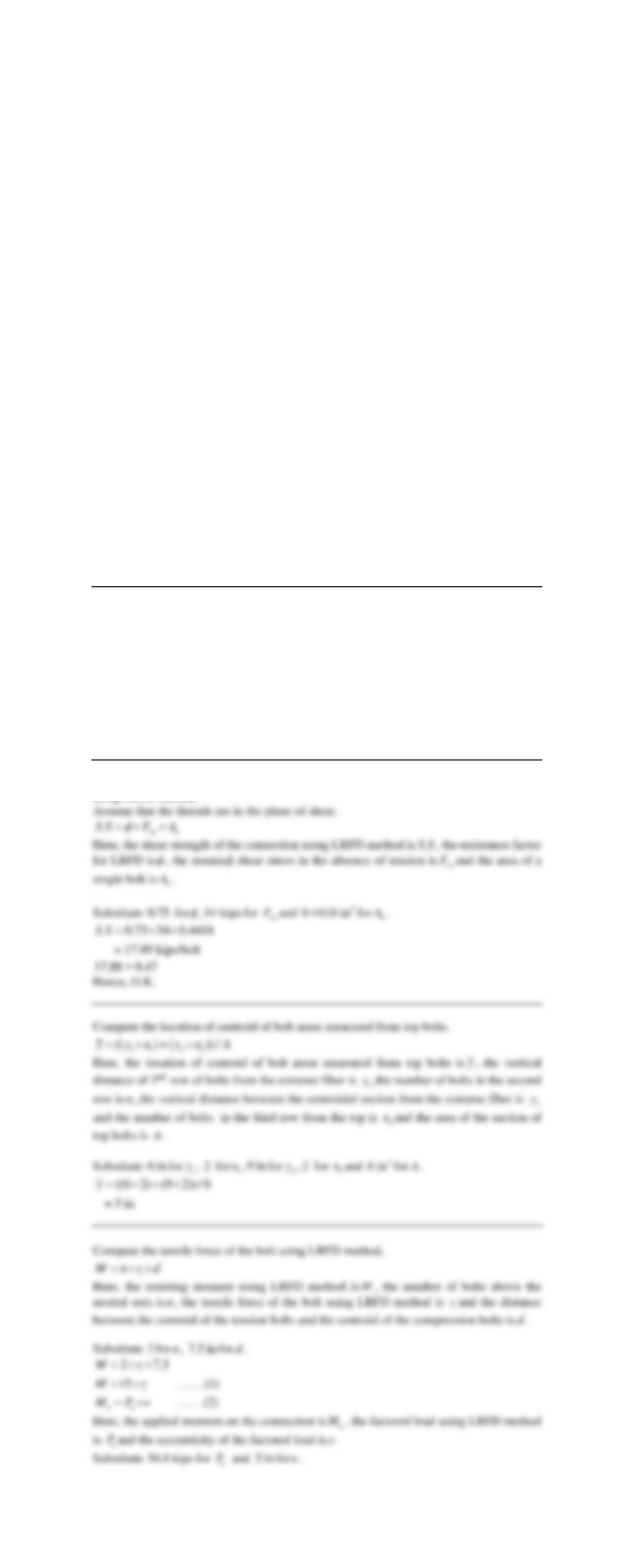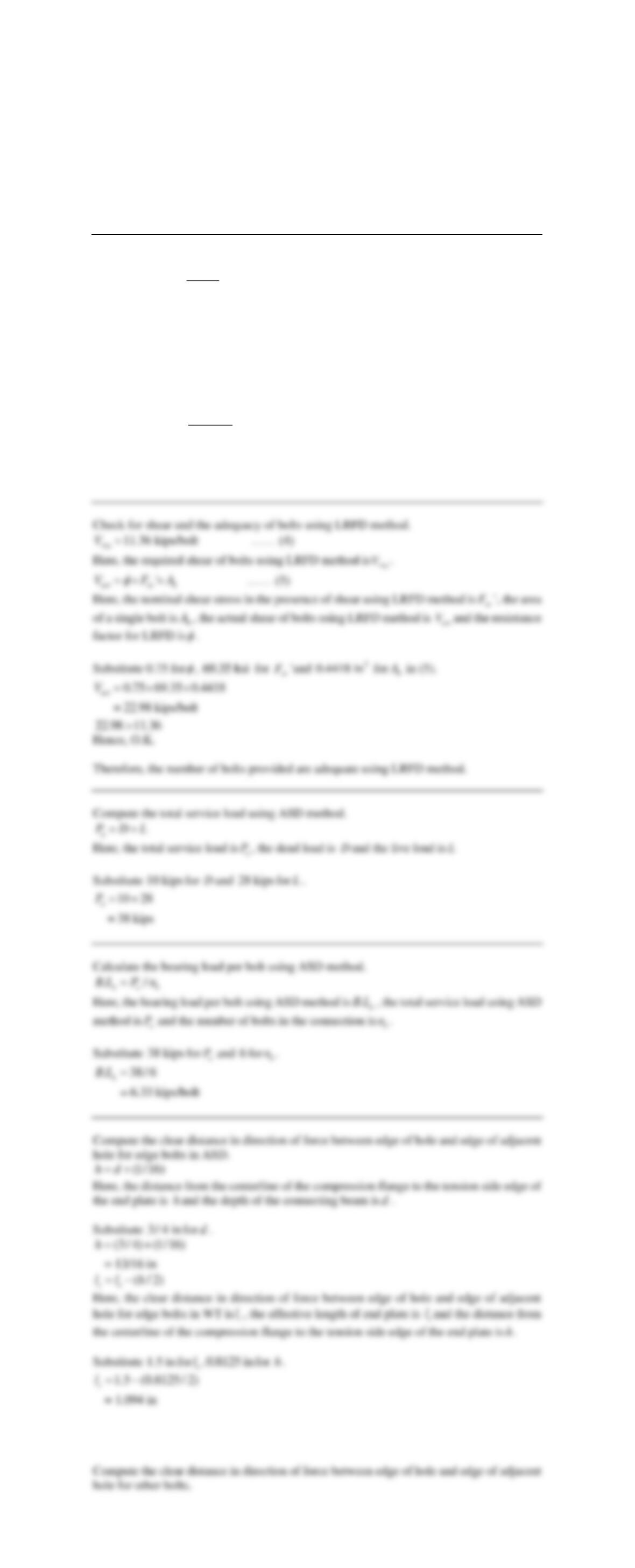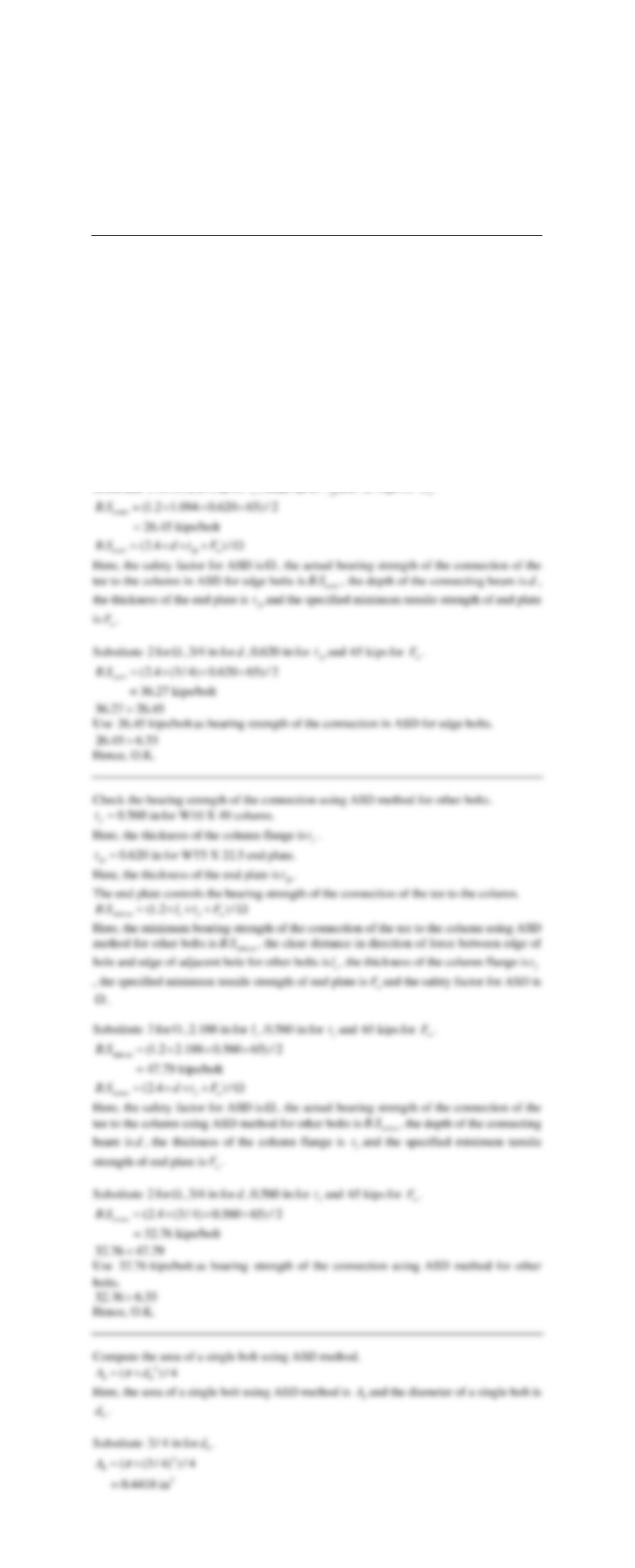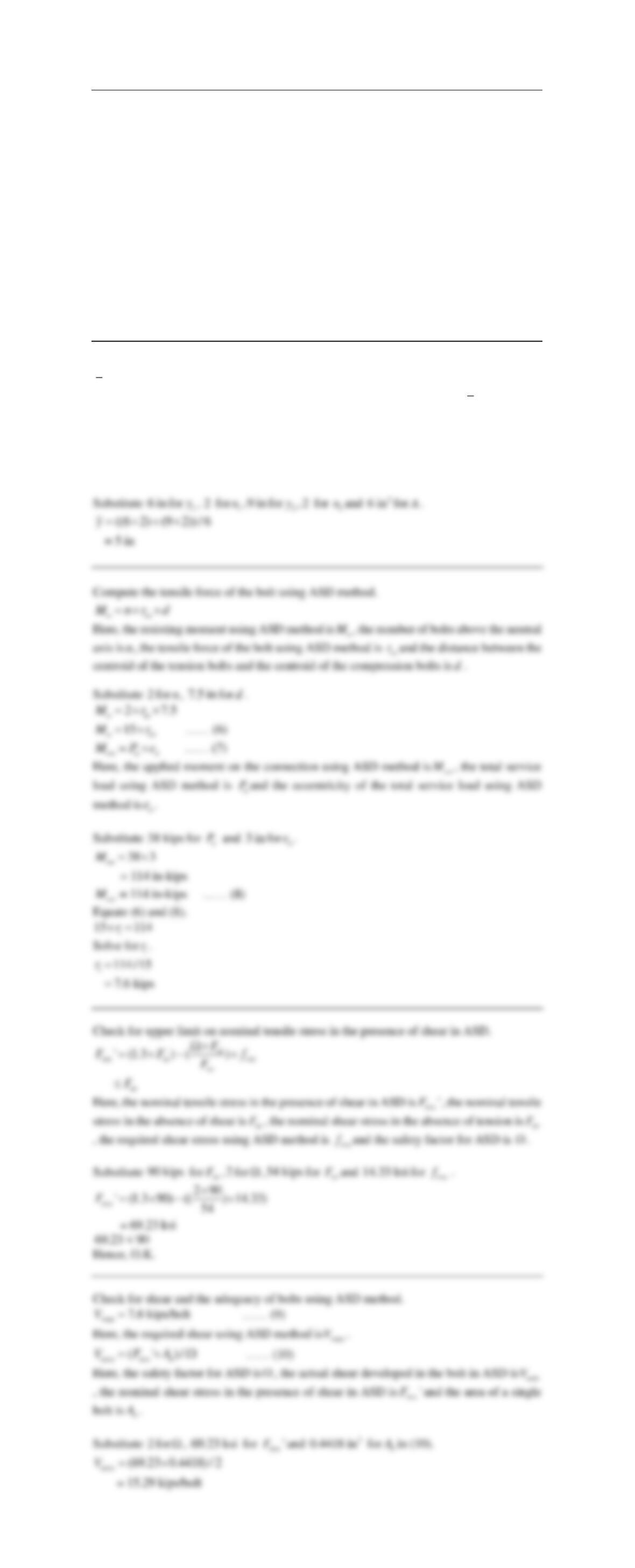Type
Essay
Pages
6 pages
Word Count
2840 words
School
steel design
Course Code
603103

# steel design

September 8, 2020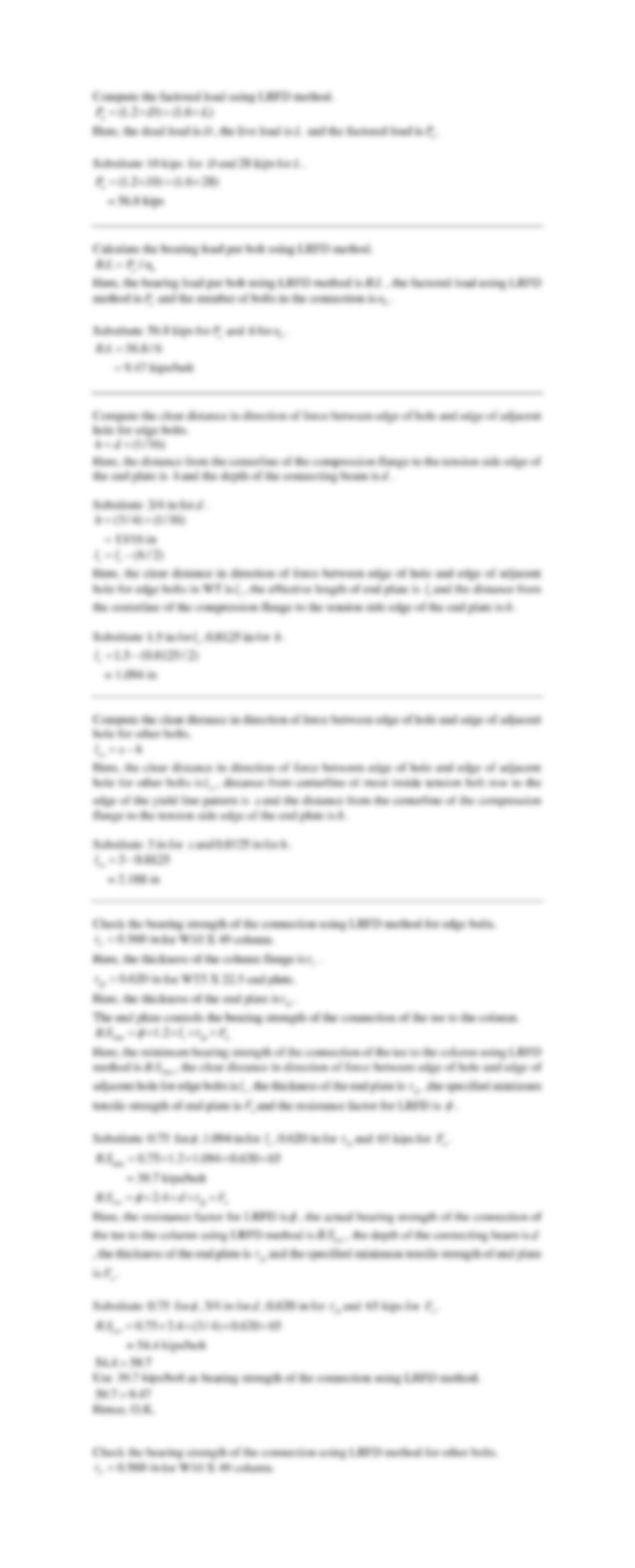Compute the factored load using LRFD method.
(1.2 ) (1.6 )
u
P D L=  +
D
L
u
P
.
Substitute
10 kips
for
D
and
28 kips
for
L
.
Calculate the bearing load per bolt using LRFD method.
./
ub
B L P n=
Here, the bearing load per bolt using LRFD method is
.BL
, the factored load using LRFD
method is
u
P
and the number of bolts in the connection is
b
n
.
Substitute
56.8 kips
for
u
P
and
6
for
b
n
.
. 56.8/ 6
= 9.47 kips/bolt
BL=
Compute the clear distance in direction of force between edge of hole and edge of adjacent
hole for edge bolts.
(1/16)hd=+
Here, the distance from the centerline of the compression flange to the tension side edge of
the end plate is
h
and the depth of the connecting beam is
d
.
Substitute
3/4 in
for
d
.
(3/ 4) (1/16)
= 13/16 in
h=+
( / 2)
ce
l l h=−
Here, the clear distance in direction of force between edge of hole and edge of adjacent
hole for edge bolts in WT is
c
l
, the effective length of end plate is
e
l
and the distance from
the centerline of the compression flange to the tension side edge of the end plate is
h
.
Substitute
1.5 in
for
e
l
,
0.8125 in
for
h
.
1.5 (0.8125/ 2)
= 1.094 in
c
l=−
Compute the clear distance in direction of force between edge of hole and edge of adjacent
hole for other bolts.
co
l s h=−
Here, the clear distance in direction of force between edge of hole and edge of adjacent
hole for other bolts is
co
l
, distance from centerline of most inside tension bolt row to the
edge of the yield line pattern is
s
and the distance from the centerline of the compression
flange to the tension side edge of the end plate is
h
.
Substitute
3 in
for
s
and
0.8125 in
for
h
.
3 0.8125
= 2.188 in
co
l=−
Check the bearing strength of the connection using LRFD method for edge bolts.
0.560 in
f
t=
for W10 X 49 column.
Here, the thickness of the column flange is
f
t
.
0.620 in
fp
t=
for WT5 X 22.5 end plate.
Here, the thickness of the end plate is
fp
t
.
The end plate controls the bearing strength of the connection of the tee to the column.
min
. 1.2 c fp u
BS l t F
=   
Here, the minimum bearing strength of the connection of the tee to the column using LRFD
method is
min
.BS
, the clear distance in direction of force between edge of hole and edge of
adjacent hole for edge bolts is
c
l
, the thickness of the end plate is
fp
t
,the specified minimum
tensile strength of end plate is
u
F
and the resistance factor for LRFD is
.
Substitute
0.75
for
,
1.094 in
for
c
l
,
0.620 in
for
fp
t
and
65 kips
for
u
F
.
min
. 0.75 1.2 1.094 0.620 65
= 39.7 kips/bolt
BS =  
f